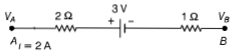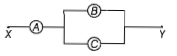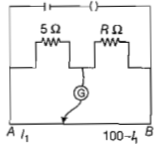A potentiometer is an accurate and versatile device to make electrical measurement of EMF because the method involves

(a) cells

(c) a condition of no current flow through the galvanometer

(d) a combination of cells, galvanometer, and resistances

Concept Questions :-

Meter bridge and potentiometer
High Yielding Test Series + Question Bank - NEET 2020

Difficulty Level:

The potential difference $\left({V}_{A}-{V}_{B}\right)$ between the points A and B in the given figure is if the current is flowing from B to A -(a) -3V                   (b) +3V

(c) +6V                  (d) +9V

Concept Questions :-

Combination of resistors
High Yielding Test Series + Question Bank - NEET 2020

Difficulty Level:

A potentiometer wire is 100 cm long and a constant potential is maintained across it. Two cells are connected in series first to support one another and then in the opposite direction. The balance points are obtained at 50 cm and 10 cm from the positive end of the wire in the two cases. The ratio of emf is

1. 5:4              2. 3:4

3. 3:2              4. 5:1

Concept Questions :-

Meter bridge and potentiometer
High Yielding Test Series + Question Bank - NEET 2020

Difficulty Level:

The charge following through a resistance R varies with time  where a and b are positive constants. The total heat produced in R is

(a) $\frac{{a}^{3}R}{3b}$               (b) $\frac{{a}^{3}R}{2b}$

(c) $\frac{{a}^{3}R}{b}$               (d) $\frac{{a}^{3}R}{6b}$

Concept Questions :-

Heating effect of current
High Yielding Test Series + Question Bank - NEET 2020

Difficulty Level:

A potentiometer wire has a length 4 m and resistance 8Ω. The resistance that must be connected in series with the wire and an accumulator of emf 2V, so as to get a potential gradient 1mV per cm of the wire is

(a)32Ω

(b)40Ω

(c)44Ω

(d)48Ω

Concept Questions :-

Meter bridge and potentiometer
High Yielding Test Series + Question Bank - NEET 2020

Difficulty Level:

A, B, and C are voltmeters of resistance R, 1.5R, and 3R respectively as shown in the figure. When some potential difference is applied between X and Y, the voltmeter readings are VA, VB, and VC respectively. Then,1. VA=VB=VC

2. VA≠VB=VC

3. VA=VB≠VC

4. VA≠VB≠VC

Concept Questions :-

Combination of resistors
High Yielding Test Series + Question Bank - NEET 2020

Difficulty Level:

Across a metallic conductor of non-uniform cross-section, a constant potential difference is applied. The quantity which remains constant along the conductor is :

1. current density
2. current
3. drift velocity
4. electric field

Concept Questions :-

Current and current density
High Yielding Test Series + Question Bank - NEET 2020

Difficulty Level:

A potentiometer wire of length L and a resistance r are connected in series with a battery of e.m.f. ${E}_{o}$ and a resistance r1. An unknown e.m.f. is balanced at a length l of the potentiometer wire. The e.m.f. E will be given by

(a)$\frac{{E}_{o}rL}{\left({r}_{1}\right)l}$

(b)$\frac{{E}_{o}rl}{\left(r+{r}_{1}\right)L}$

(c)Eol/L

(d)$\frac{{E}_{o}rL}{\left(r+{r}_{1}\right)l}$

Concept Questions :-

Meter bridge and potentiometer
High Yielding Test Series + Question Bank - NEET 2020

Difficulty Level:

Two cities are 150 km apart. Electric power is sent from one city to another city through copper wires. The fall of potential per km is 8V and the  average resistance per km is 0.5 Ω. The power loss in the wire is

(a) 19.2W

(b) 19.2kW

(c) 19.2J

(d) 12.2kW

Concept Questions :-

Heating effect of current
High Yielding Test Series + Question Bank - NEET 2020

Difficulty Level:

The resistances in the two arms of the meter bridge are 5 and R , respectively. When the resistance R is shunted with an equal resistance, the new balance point is at 1.6${l}_{1}$. The resistance R, is(a)10Ω

(b)15Ω

(c)20Ω

(d)25Ω